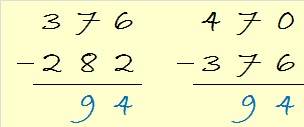# 470 Greatest Common Factors of Pythagorean Triples.

• 470 is a composite number.
• Prime factorization: 470 = 2 x 5 x 47
• The exponents in the prime factorization are 1, 1, and 1. Adding one to each and multiplying we get (1 + 1)(1 + 1)(1 + 1) = 2 x 2 x 2 = 8. Therefore 470 has exactly 8 factors.
• Factors of 470: 1, 2, 5, 10, 47, 94, 235, 470
• Factor pairs: 470 = 1 x 470, 2 x 235, 5 x 94, or 10 x 47
• 470 has no square factors that allow its square root to be simplified. √470 ≈ 21.67948—————————————————————————————-

470 is the hypotenuse of the non-primitive Pythagorean triple 282-376-470. What is the greatest common factor of those three numbers?

The greatest common factor will always be a factor of the smallest of the three numbers, but it will also be a factor of the smallest difference between the three numbers as well. Let’s find those differences. Note: the difference between the 282 and 470 will not be the smallest difference so there is no need to find that one. We only need to find these two differences:In the case of this Pythagorean triple the differences are equal to each other which means that the difference, 94*, is also the greatest common factor of the three numbers! Go ahead and try dividing each number in the triple by 94. You will discover that this Pythagorean triple is just 3-4-5 multiplied by 94.

*This statement is only true of Pythagorean triples. For example the following numbers also have differences of 94, but the greatest common factor is not 94, but a factor of 94:

1. The greatest common factor of 283-377-471 is 1.
2. The greatest common factor of 284-378-472 is 2
3. The greatest common factor of 329-423-517 is 47

Mathchat has written an excellent post on finding the greatest common factor of three or more numbers that can be used for all integers in general.

But as far as Pythagorean triples are concerned, anytime the corresponding differences of a Pythagorean triple are equal to each other, then that Pythagorean triple is just 3-4-5 multiplied by the difference. There are an infinite number of such triples, and 282-376-470 is just one of them.Now remember there is an infinite number of primitive Pythagorean triples, and every one of those triples can be multiplied by each of the infinitely many counting numbers. A graphic like the one above could be made for every primitive triple followed by each of its multiples. For example 5-12-13, 10-24-26, 15- 36-39, etc. would be another infinite series of Pythagorean triples.

You could say the total number of Pythagorean triples equals infinity times infinity!

## 4 thoughts on “470 Greatest Common Factors of Pythagorean Triples.”

1.abyssbrain

When I need to find the GCF of some numbers, I normally use the Euclidean algorithm (you know, the one used for solving linear Diophantine equations), especially if the numbers are large, since it’s very efficient.

•ivasallay

I’m not familiar with that algorithm. If it isn’t too complicated, perhaps you could write a post about it.

•abyssbrain

Though the proof of the algorithm and the algorithm’s other applications can be quite rigorous, its most basic application (for finding GCF) is actually pretty easy. I’ll make a post about it later.

•ivasallay

I look forward to seeing how you apply your mathemagic to this important concept!

This site uses Akismet to reduce spam. Learn how your comment data is processed.